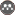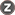Volume 9, Issue 1 (Jan-Mar 2020)                   JCHR 2020, 9(1): 1-3 | Back to browse issues page

BibTeX | RIS | EndNote | Medlars | ProCite | Reference Manager | RefWorks
Send citation to:Taherzadeh Chenani K, Madadizadeh F. Popular Statistical Tests for Investigating the Relationship between Two Variables in Medical Research. JCHR. 2020; 9 (1) :1-3
URL: http://jhr.ssu.ac.ir/article-1-619-en.html
1- Department of Biostatistics and Epidemiology, School of Public Health, Shahid Sadoughi University of Medical Sciences, Yazd, Iran
Full-Text:   (329 Views)

Dear Editor in Chief,
Recently, using statistical tests as useful and ubiquitous tools in medical data analysis is increasing (1). However, some researchers are using statistical methods without sufficient knowledge. The purpose of this letter is to provide applied information on some of the most commonly used statistical tests.
All statistical tests examine a hypothesis. A hypothesis is defined as a claim that its accuracy or inaccuracy is unknown (2). In general, variables are divided into quantitative and qualitative categories (3). Some popular statistical tests that examine the relationship between two or more variables are summarized bellow according to the main purpose of this article.
• Investigating the linear relationship between two quantitative variables: correlation coefficient test
The correlation coefficient test examines the linear relationship between two variables. The null hypothesis in this test is the absence of a linear relationship (4). Before examining the relationship between two quantitative variables, it is necessary to examine the normality of the error distribution in the quantitative variables through normality
tests such as the Kolmogorov-Smirnov and Shapiro-Wilk tests (5). The null hypothesis in the normality tests is that the distribution of errors in the variable is normal. If the null hypothesis is rejected (p value<significant level), it means that the distribution of errors in the variable is non normal (5). After the normality check of the errors in the variables, if both variables had a normal distribution, Pearson's correlation coefficient would be a good choice and Spearman correlation coefficient would be used if one of the two variables had non normal distribution (6).
• Investigating the relationship between two qualitative variables: Chi-square test
The null hypothesis in Chi-square test indicates that two qualitative variables are independent. In other words, this test examines whether the frequency of the observations at each of the composite levels is the same or not. If the expected frequency at any combination level is less than 5, Fisher's exact test will be replaced with that (3). Fisher's exact test is used to compare ratios or the prevalence of two independent groups (usually in 2×2 contingency table). If the two groups are not independent, McNemar’s test will be used (7).
• Investigating the relationship between a quantitative variable and a two independent-level qualitative variable: Independent-Samples T-test and Mann-Whitney U test
If the qualitative variable has two levels with independent samples in each level, to investigate the relation with a quantitative variable Independent-Samples T-test and Mann-Whitney U test are used (7).
Independent-Samples T-Test compares the mean of a quantitative variable during two independent level of a qualitative variable. The null hypothesis in this test is the mean equality during the qualitative variable levels (4). If the data are low (less than 20) or the distribution of errors in the quantitative variable does not follow the normal distribution, the nonparametric Mann-Whitney U test will be replaced comparing the median of the quantitative variables in two groups. In reporting nonparametric test results instead of the mean and standard deviation, median and interquartile range (IQR) are used (4).
• Investigating the relationship between a quantitative variable and a two dependent -level qualitative variable (before-after): paired Samples T-test and Wilcoxon signed-rank test
If the qualitative variable levels are before-after or first and second times measuring, to investigating the relation with a quantitative variable the paired (or dependent) Samples T-test   would be appropriate and if the data is low (less than 20) or the distribution of errors in the quantitative variable does not follow the normal distribution, the alternative test will be the Wilcoxon signed-rank test. The Wilcoxon signed-rank test is a nonparametric test that compares the median of the quantitative variables during two levels of the qualitative variable (4, 7).
• Investigating the relationship between a quantitative variable and a qualitative variable with more than two independent levels: one-way
ANOVA or Kruskal-Wallis test
If the qualitative variable has more than two levels, to investigating the relation with a quantitative variable   one-way analysis of variance (one way-ANOVA) or Kruskal-Wallis tests are used for independent levels (3).
One-way ANOVA test investigates the relationship between a more than two independent -level qualitative variable with a quantitative variable when the null hypothesis in this test means the equality of the mean of the quantitative variable in different levels of the qualitative variable (3, 4, and 7). If the data is low (less than 20) or the distribution of errors in the variable does not follow the normal distribution, the alternative nonparametric test will be the Kruskal-Wallis
test. Kruskal-Wallis test also compares the median of quantitative variables at qualitative variable levels (7).
• Investigating the relationship between a quantitative variable and a qualitative variable with more than two dependent levels: one-way repeated measures ANOVA or Friedman test
If the levels of qualitative variables were before, after, or time of measuring such as first, second, and etc., one-way repeated measures analysis of variance (ANOVA) test with null hypothesis equality of quantitative variable means at the qualitative variable levels would be a good choice (4). If the data is low (less than 20) or the distribution of errors in the quantitative variable does not follow the normal distribution, the alternative test will be the Friedman nonparametric test. The Friedman test compares the median of the quantitative variable at the qualitative variable levels (7).
Each statistical test has its place and application. Medical researchers with knowing the proper use of statistical tests, can improve the quality of their research; therefore, they can improve the health and medical status of the community.
Review: Letter to The Editor | Subject: Epidemiology
Received: 2020/03/16 | Accepted: 2020/04/5 | Published: 2020/03/29

References
1. Madadizadeh F, Asar ME, Hosseini M. Common Statistical Mistakes in Descriptive Statistics Reports of Normal and Non-Normal Variables in Biomedical Sciences Re‌ search. Iranian journal of public health. 2015;44(11):1557-8.
2. Larner A. Diagnostic test accuracy studies in dementia: a pragmatic approach: Springer; 2019. [DOI:10.1007/978-3-030-17562-7]
3. Madadizadeh F, Bahrampour A, Mousavi SM, et al. Using Advanced Statistical Models to Predict the Non-Communica‌ ble Diseases. Iranian journal of public health. 2015;44(12):1714-5.
4. Tronstad C, Pripp AH. Statistical methods for bioimpedance analysis. Journal of Electrical Bioimpedance. 2019;5(1):14-27. [DOI:10.5617/jeb.830]
5. Mishra P, Pandey CM, Singh U, et al. Descriptive statistics and normality tests for statistical data. Annals of cardiac anaesthesia. 2019;22(1):67. [DOI:10.4103/aca.ACA_157_18]
6. Porter AM. Misuse of correlation and regression in three medical journals. Journal of the Royal Society of Medicine. 1999;92(3):123-8. [DOI:10.1177/014107689909200306]
7. Petrie A, Sabin C. Medical statistics at a glance: John Wiley & Sons; 2019.Rights and permissionsThis work is licensed under a Creative Commons Attribution-NonCommercial 4.0 International License.